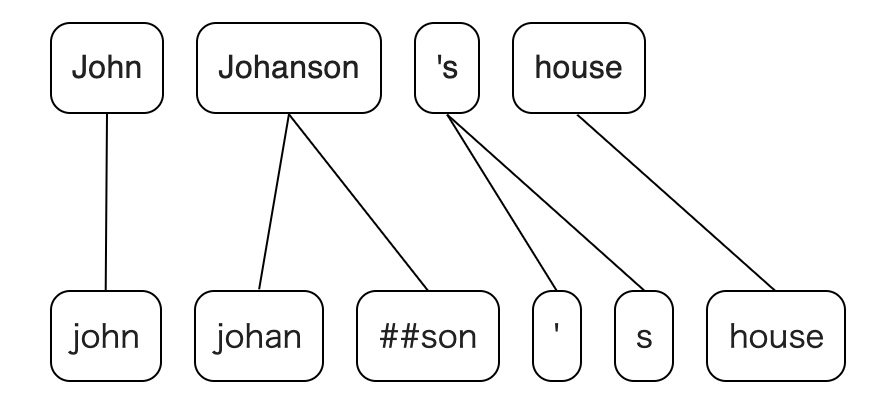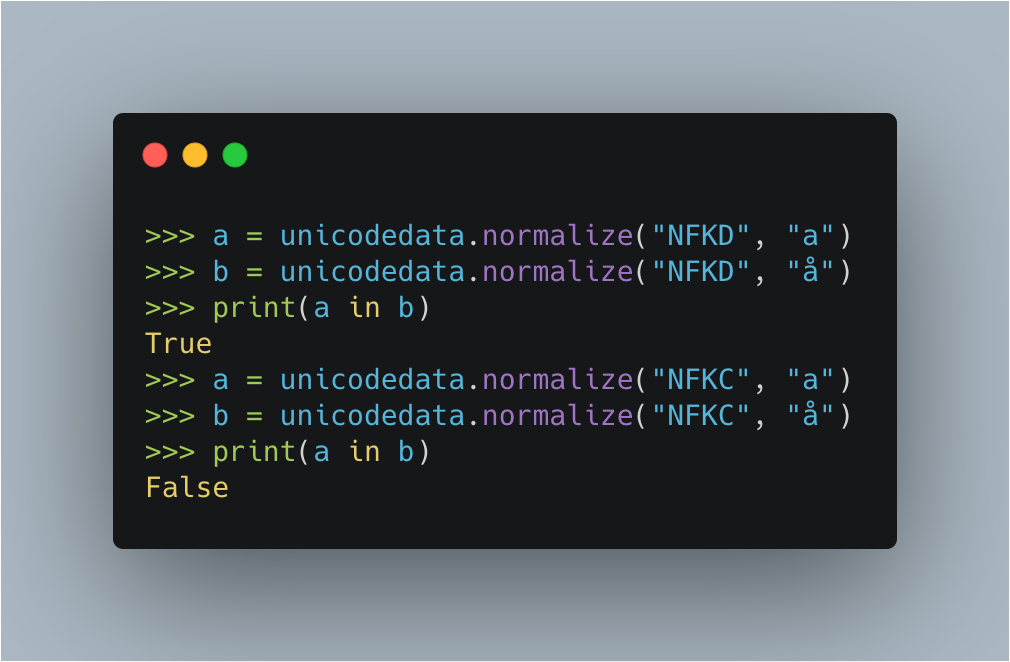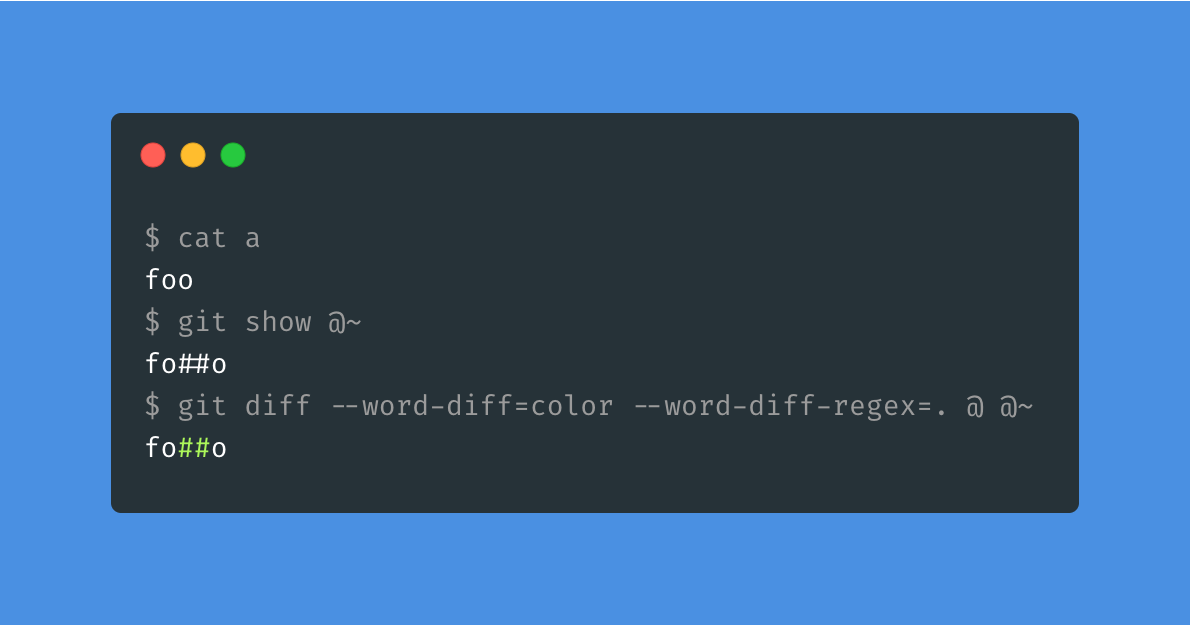Instantly share code, notes, and snippets.

# tamuhey/tokenizations_post.md

Last active March 11, 2023 05:00
How to calculate the alignment between BERT and spaCy tokens effectively and robustly

# How to calculate the alignment between BERT and spaCy tokens effectively and robustlyNatural Language Processing (NLP) has made great progress in recent years because of neural networks, which allows us to solve various tasks with end-to-end architecture. However, many NLP systems still require language-specific pre- and post-processing, especially in tokenizations. In this article, I describe an algorithm that simplifies calculating correspondence between tokens (e.g. BERT vs. spaCy), one such process. And I introduce Python and Rust libraries that implement this algorithm. Here are the library and the demo site links:

# What is "alignment" of tokens and Why is it necessary?

Suppose we want to combine a BERT-based named entity recognition (NER) model with a rule-based NER model built on top of spaCy. Although BERT's NER exhibits extremely high performance, it is usually combined with rule-based approaches for practical purposes. In such cases, what often bothers us is that tokens of spaCy and BERT are different, even if the input sentences are the same. For example, let's say the input sentence is "John Johanson 's house"; BERT tokenizes this sentence like `["john", "johan", "##son", "'", "s", "house"]` and spaCy tokenizes it like `["John", "Johanson", "'s", "house"]`. To combine the outputs, we need to calculate the correspondence between the two different token sequences. This correspondence is the "alignment".

# How to compute the alignment?

First, let's sort out the problem. Looking at the previous example, it can be said that two different token sequences have the following characteristics:

1. Splitted in different offsets
2. Normalized (e.g. lowercase, Unicode normalization, dropping accents...)
3. Added noise (meta symbol '#' in the previous case)

If the token sequences differ only in 1., it can be easily solved, because we just need to compare the letters in order from the beginning. In fact, `spacy.gold.align`, which I implemented previously, is based on this algorithm.

However, when the features 2. and 3. are taken into account, the problem suddenly becomes more difficult. If you want to deal with the previous example, it is relatively easily solved by lowercasing (e.g. A -> a) and removing meta symbols (e.g. "#" -> ""), but this depends on each tokenizer and isn't generally useful. Of course, we want a generic implementation that works for any tokenizers.

Let's think about how to deal with 2. and 3..

## Normalization

In order to compare letters, we need to normalize the input tokens at first. This is because even though two letters may look the same, the underlying data may be different. There are variety of normalization methods which is used in NLP. For example:

Unicode normalizations are defined in Unicode Standard. There are 4 types of Unicode normalizations: NFC, NFD, NFKC, NFKD. Of these, in NFKD, letters are decomposed based on compatibility, and the number of letter types are the least and the probability of matching is highest among the four methods. (see Unicode document for detail). For example, you can detect the letter "a" is a part of "å" with NFKD, but not with NFKC.Thus, we first normalize the intput tokens in NFKD form. Then, we lowercase all letters because lowercasing is also often used in NLP.

## Compare noisy texts

Now we can compare almost all tokens thanks to NFKD and lowercasing, but they still contain some noise (e.g. "#"), so we cannot completely compare all letters in tokens. How to properly ignore the noises and compare all letters? I racked my brain for few days trying to solve this problem.

Then, I came up with a solution based on a tool that I use every day. It is diff. diff is a tool that compares two texts and outputs the mismatches. It is built in `git` as `git diff`, and you can display the charcter-level correspondence as follows:In our case, what we want to know is the agreement part, not the difference, but these are pretty much the same thing. So, what kind of algorithms is `diff` based on?

According to the git diff documentation, it is based on Myers' algorithm. Myers' algorithm is one of the dynamic programming methods that computes the shortest path of what is called edit graph. It works very fast especially if the difference of the two inputs is small. For now, what we want to compare are almost identical, so we can get the correspondence of the letters very quickly.

In short, it turns out that Myers' algorithm helps us to get the correspondens of the letters in two sequence of tokens, while properly ignoring some noises.

## Overview of the algorithm

The considerations so far have shown that suitale normalizations and character-based diff gives us a generic method for computing the alignment of two token sequences. Let's summarize the specific steps briefly.

Let `tokens_a` and `tokens_b` be token sequences of type `List[str]` to be compared. For example, `tokens_a = ["foo", "bar", "baz"]`.

1. Normalize all tokens with `NFKD` and lowercasing.

For example, `"Foo" -> "foo"`

1. Concatenate the tokens into one string and let the results be `cat_a` and `cat_b` respectively.

For example, `cat_a = "".join(tokens_a)` in Python.

1. Get the character based diff between the strings `cat_a` and `cat_b`.

The character based diff can be calculated with Myers' algorithm.

1. Converts the caracter-based diff to a token-based diff.

This is relatively easy to calculate because we know the mapping between the characters and tokens in step 2.

# Implementation

Here is the repository that implements this algorithm. This library, `tokenizations`, is implemented with Rust and provides a Python binding.

For example, you can use the Python library as follows:

```# `\$ pip install pytokenizations` to install the package
import tokenizations

tokens_a = ["John", "Johanson", "'s", "house"]
tokens_b = ["john", "johan", "##son", "'", "s", "house"]
a2b, b2a = tokenizations.get_alignments(tokens_a, tokens_b)

for i in range(len(tokens_a)):
print(tokens_a[i])
for j in a2b[i]:
print("    ", tokens_b[j])```
``````John
john
Johanson
johan
##son
's
'
s
house
house
``````

# Conclusion

In this article, I introduced an algorithm to align two token sequences that are produced by two different tokenizers. The title mentions spaCy and BERT, but this algorithm can be applied to any tokenizers. Also, it can be useful to apply NLP methods to noisy texts which contains HTML tags for example: remove the tags, apply the methods, then calculate the alignment for the output and original text. Here are the links to the library and demo.